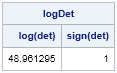A few years ago I wrote an article that shows how to compute the log-determinant of a covariance matrix in SAS. This computation is often required to evaluate a log-likelihood function. My algorithm used the ROOT function in SAS/IML to compute a Cholesky decomposition of the covariance matrix. The Cholesky decomposition exists only for symmetric positive definite matrices, which means that this implementation cannot be used to compute the log-determinant of an arbitrary square matrix.

For an arbitrary square matrix, the determinant can be negative or zero. The log function is undefined for nonpositive values, but the log of the absolute value of the determinant always exists. This computation, along with the sign of the determinant, enables you to reconstruct the determinant of any matrix.

SAS/IML 13.1 supports the LOGABSDET function, which computes the logarithm of the absolute value of the determinant for any square matrix. The function returns a vector with two elements: the first element is the logarithm, the second is the sign of the determinant.

In my earlier article I wrote:

The determinant of a Vandermonde matrix with consecutive integer elements increases super-factorially with the dimension of the matrix! For a 30 x 30 integer Vandermonde matrix, the determinant is too large to represent as a standard double-precision floating-point number.

A Vandermonde matrix is not symmetric, so let's construct a Vandermonde matrix and compute its log-determinant by using the LOGABSDET function. The following SAS/IML function computes a Vandermonde matrix as defined in Golub and van Loan. The Wikipedia article uses the transpose of this matrix as the definition.

```proc iml; /* Given a vector v = {v1 v2 v3 ... vn}, this function returns the Vandermonde matrix defined by [ 1 1 1 ... 1 ] [ v1 v2 v3 ... vn ] [ v1^2 v2^2 v3^2 ... vn^2 ] [ ... ... ... ... ... ] [ v1^{n-1} ... ... vn^{n-1} ] */ start CreateVandermondeMatrix( _v ); v = rowvec(_v); m = ncol(v); mVander = j(m,m,1); do i = 2 to m; mVander[i,] = mVander[i-1,] # v; end; return ( mVander ); finish;   v = 1:10; m = CreateVandermondeMatrix(v); logDet = logabsdet(m); print logDet[colname={"log(det)" "sign(det)"}];```For this 1 x 10 matrix, the log-determinant is about 49, which means that the determinant is exp(49)  ≈ 1.8 x 1021. For the 30 x 30 nonsymmetric matrix generated from v = 1:30, the determinant is too large to be computed by using the DET function, but the LOGABSDET function has no problem computing the log-determinant, which is approximately 901.

Share